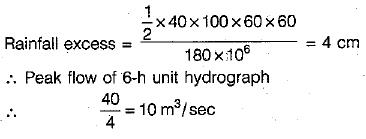Courses

# Hydrographs - 1

## 10 Questions MCQ Test GATE Civil Engineering (CE) 2022 Mock Test Series | Hydrographs - 1

Description
This mock test of Hydrographs - 1 for Civil Engineering (CE) helps you for every Civil Engineering (CE) entrance exam. This contains 10 Multiple Choice Questions for Civil Engineering (CE) Hydrographs - 1 (mcq) to study with solutions a complete question bank. The solved questions answers in this Hydrographs - 1 quiz give you a good mix of easy questions and tough questions. Civil Engineering (CE) students definitely take this Hydrographs - 1 exercise for a better result in the exam. You can find other Hydrographs - 1 extra questions, long questions & short questions for Civil Engineering (CE) on EduRev as well by searching above.
QUESTION: 1

### The recession limb of a flood hydrograph can be expressed with positive values of coefficients, as Qt/Q0 =

Solution:

Barnes showed that recession limp of a flood hydrograph can be expressed as,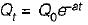where a = -In kr
kr is recession constant of value less than unity. Q0 and Qt are discharges at a time interval of t days with Q0 being the initial discharge.

QUESTION: 2

### For a given storm, other factors remaining same,

Solution:

The drainage density is defined as the ratio of the total channel length to the total drainage area. A large drainage density creates situation conductive for quick disposal of runoff drawn the channels.

QUESTION: 3

### Base-flow separation is performed

Solution:
QUESTION: 4

A unit hydrograph has

Solution:
QUESTION: 5

The basic assumptions of the unit-hydrograph theory are

Solution:
QUESTION: 6

The D-hour unit hydrograph of a catchment may be obtained by dividing the ordinates of a single peak Direct Runoff Hydrograph (DRH) due to a storm of D-hour duration by the

Solution:
QUESTION: 7

A triangular DRH due to a storm has a time base of 80 hrs and a peak flow of 50 m3/s occurring at 20 hours from the start, if the catchment area is 144 km2, the rainfall excess in the storm was

Solution: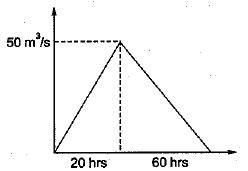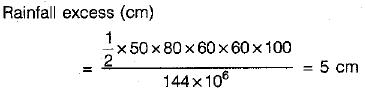QUESTION: 8

A 12-hr unit hydrograph of a catchment is triangular in shape with a base width of 144 hours and a peak discharge value of 23 m3/s. This unit hydrograph refers to a catchment of area

Solution: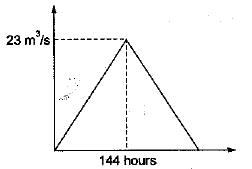Unit hydrograph refers to 1 cm of rainfall excess
∴ Let area of catchment = A(km2)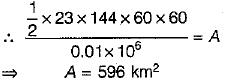QUESTION: 9

A 90 km2 catchment has a 4-h unit hydrograph which can be approximated as a triangle. If the peak ordinate of this unit hydrograph is 10m3/s the time base is

Solution:

Let the time base = B(hours)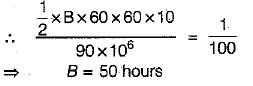QUESTION: 10

A triangular DRH due to a 6-h storm in a catchment has a time base of 100 h and a peak flow of 40 m3/s. The catchment area is 180 km2. The 6-h unit hydrograph of this catchment will have a peak flow in m3/s of

Solution: